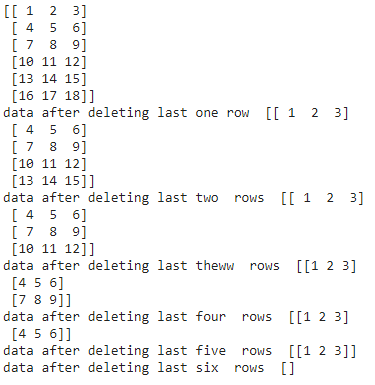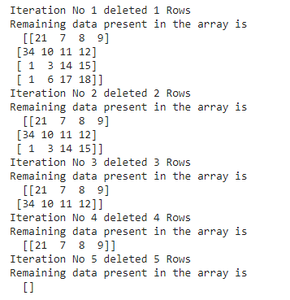How to delete last N rows from Numpy array?

• Last Updated : 28 Apr, 2021

In this article, we will discuss how to delete the last N rows from the NumPy array.

Method 1: Using Slice Operator

Slicing is an indexing operation that is used to iterate over an array.

Syntax: array_name[start:stop]

where start is the start is the index and stop is the last index.

We can also do negative slicing in Python. It is denoted by the below syntax.

Syntax: array_name[: -n]

where, n is the number of rows from last to be deleted.

Example1:

We are going to create an array with 6 rows and 3 columns and delete last N rows using slicing.

Python3

 # importing numpy moduleimport numpy as np  # create an array with 6 rows and 3 columnsa = np.array([[1, 2, 3], [4, 5, 6], [7, 8, 9],               [10, 11, 12], [13, 14, 15], [16, 17, 18]])  print(a)  # delete last 1 st rowprint("data after deleting last one row ", a[:-1])  # delete last 2 nd  rowprint("data after deleting last two  rows ", a[:-2])  # delete last 3 rd  rowprint("data after deleting last theww  rows ", a[:-3])  # delete last 4 th  rowprint("data after deleting last four  rows ", a[:-4])  # delete last 5 th  rowprint("data after deleting last five  rows ", a[:-5])  # delete last 6 th  rowprint("data after deleting last six  rows ", a[:-6])

Output:Example 2:

We use for loop to iterate over the elements and use the slice operator, we are going to delete the data and then print the data.

Python3

 # importing numpy moduleimport numpy as np  # create an array with 5 rows and # 4 columnsa = np.array([[21, 7, 8, 9], [34, 10, 11, 12],               [1, 3, 14, 15], [1, 6, 17, 18],               [4, 5, 6, 7]])  # use for loop to iterate over the# elementsfor i in range(1, len(a)+1):    print("Iteration No", i, "deleted", i, "Rows")    print("Remaining data present in the array is\n ", a[:-i])

Output:Example 3:

We can also specify the elements that we need and store them into another array variable using the slice operator. In this way, we will not get the last N rows (delete those).

Python3

 # importing numpy moduleimport numpy as np  # create an array with 5 rows and # 4 columnsa = np.array([[21, 7, 8, 9], [34, 10, 11, 12],               [1, 3, 14, 15], [1, 6, 17, 18],              [4, 5, 6, 7]])  # place first 2 rows in b variable # using slice operatorb = a[:2]  print(b)

Output:

[[21  7  8  9]
[34 10 11 12]]

Method 2: Using numpy.delete() method

It is used to delete the elements in a NumPy array based on the row number.

Syntax: numpy.delete(array_name,[rownumber1,rownumber2,.,rownumber n],axis)

Parameters:

• array_name is the name of the array.
• row numbers is the row values
• axis specifies row or column
• axis=0 specifies row
• axis=1 specifies column

Here we are going to delete the last rows so specify the rows numbers in the list.

Example 1: Delete last three rows

Python3

 # importing numpy moduleimport numpy as np  # create an array with 5 rows and # 4 columnsa = np.array([[21, 7, 8, 9], [34, 10, 11, 12],               [1, 3, 14, 15], [1, 6, 17, 18],               [4, 5, 6, 7]])  # delete last three rows# using numpy.deletea = np.delete(a, [2, 3, 4], 0)print(a)

Output:

[[21  7  8  9]
[34 10 11 12]]

Example 2: Delete all rows

Python3

 # importing numpy moduleimport numpy as np  # create an array with 5 rows and 4 columnsa = np.array([[21, 7, 8, 9], [34, 10, 11, 12],               [1, 3, 14, 15], [1, 6, 17, 18],               [4, 5, 6, 7]])  # delete last three rows# using numpy.deletea = np.delete(a, [0, 1, 2, 3, 4], 0)print(a)

Output:

[ ]

Attention geek! Strengthen your foundations with the Python Programming Foundation Course and learn the basics.

To begin with, your interview preparations Enhance your Data Structures concepts with the Python DS Course. And to begin with your Machine Learning Journey, join the Machine Learning - Basic Level Course

My Personal Notes arrow_drop_up